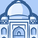# QlikView App Dev

Discussion Board for collaboration related to QlikView App Development.

Announcements
Action-Packed Learning Awaits! QlikWorld 2023. April 17 - 20 in Las Vegas: REGISTER NOW
cancel
Showing results for
Did you mean:Contributor III

## SUM IF

Hello,

I have the following expression

=sum(if((IDEFAC='L'),MFAC))

It's works fine, now I want to add for value IDEFAC 'L' and  'N' and 'S' and 'F'

I try this expression

=sum(if((IDEFAC='L' and IDEFAC='N' and IDEFAC='S' and IDEFAC='F'),MFAC)) but it's not works

Someone have an idea?

1 Solution

Accepted SolutionsEmployee

But if you insist using IF

=sum(if((IDEFAC='L' OR IDEFAC='N' OR IDEFAC='S' OR IDEFAC='F'),MFAC))

6 RepliesEmployee

Maybe using Set Analysis makes things easier

=sum({<IDEFAC={'L','N','S','F'>}MFAC))Employee

But if you insist using IF

=sum(if((IDEFAC='L' OR IDEFAC='N' OR IDEFAC='S' OR IDEFAC='F'),MFAC))MVP

SUM({<Customer_Name = {'A','B','C','D'}>}QTY)MVP

Hi

Try like this

=sum({<IDEFAC={'L','N','S','F'>} MFAC) // or function

or

=sum({<IDEFAC={'L'}> * <IDEFAC={'N'}> * <IDEFAC={'S'}> * <IDEFAC={'F'}>} MFAC) // And Function

Thanks & Regards,
Mayil Vahanan RContributor III
Author

Thank you Clever,

Expression under works fine

=sum(if((IDEFAC='L' OR IDEFAC='N' OR IDEFAC='S' OR IDEFAC='F'),MFAC))MVP

FYI .... IF is much slower compare to SET ANALYSIS.Community Browser